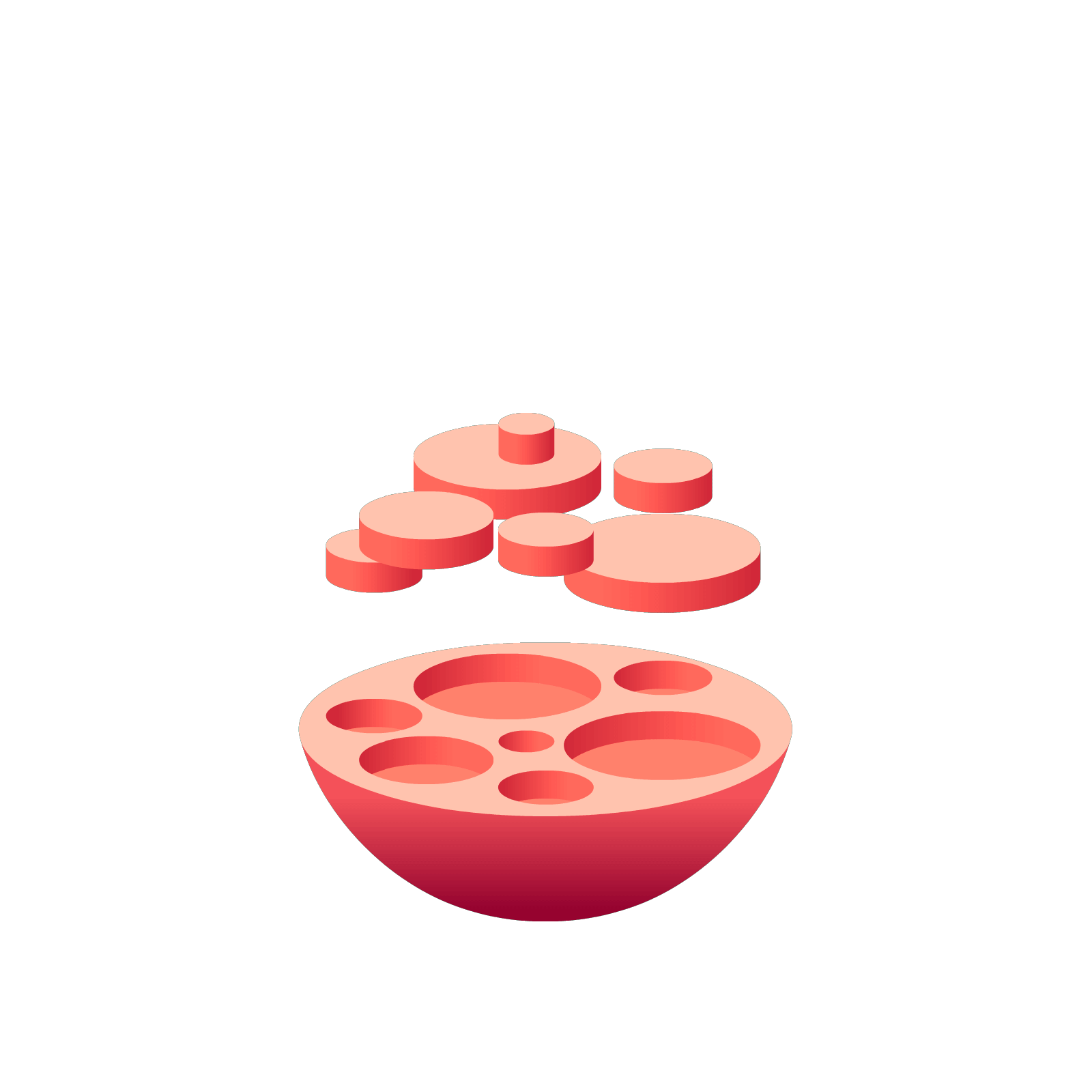06 285 000# Station  10SubtractionLet's use the antidots we've just created to examine subtraction.

### Question 1

Consider this subtraction problem.To me, this is really $536$ plus the opposite of $123$.
That is, we're adding one anti-hundred, two anti-tens, and three anti-ones to five hundreds, three tens and six ones.

Put the numbers $536$ and $-123$ into a $1\leftarrow 10$ machine.### Question 2

Make the annihilations required to show the value of $413$.### Question 3

Try this problem on the machine!### Question 4

Unexplode some dots to clarify the result of the subtraction.### Question 5

Here’s a very long subtraction problem. Compute it the dots and antidots way and then fix up your answer for society’s sake.

Thinking Question: As you fix up your answer for society, does it seem easier to unexplode from left to right or from right to left?All correct approaches to mathematics are correct – even this strange approach to subtraction. Actually, if you click on the station below about the Traditional Algorithm, you will see my approach here isn’t actually that new or different!

### Let's Go Wild!